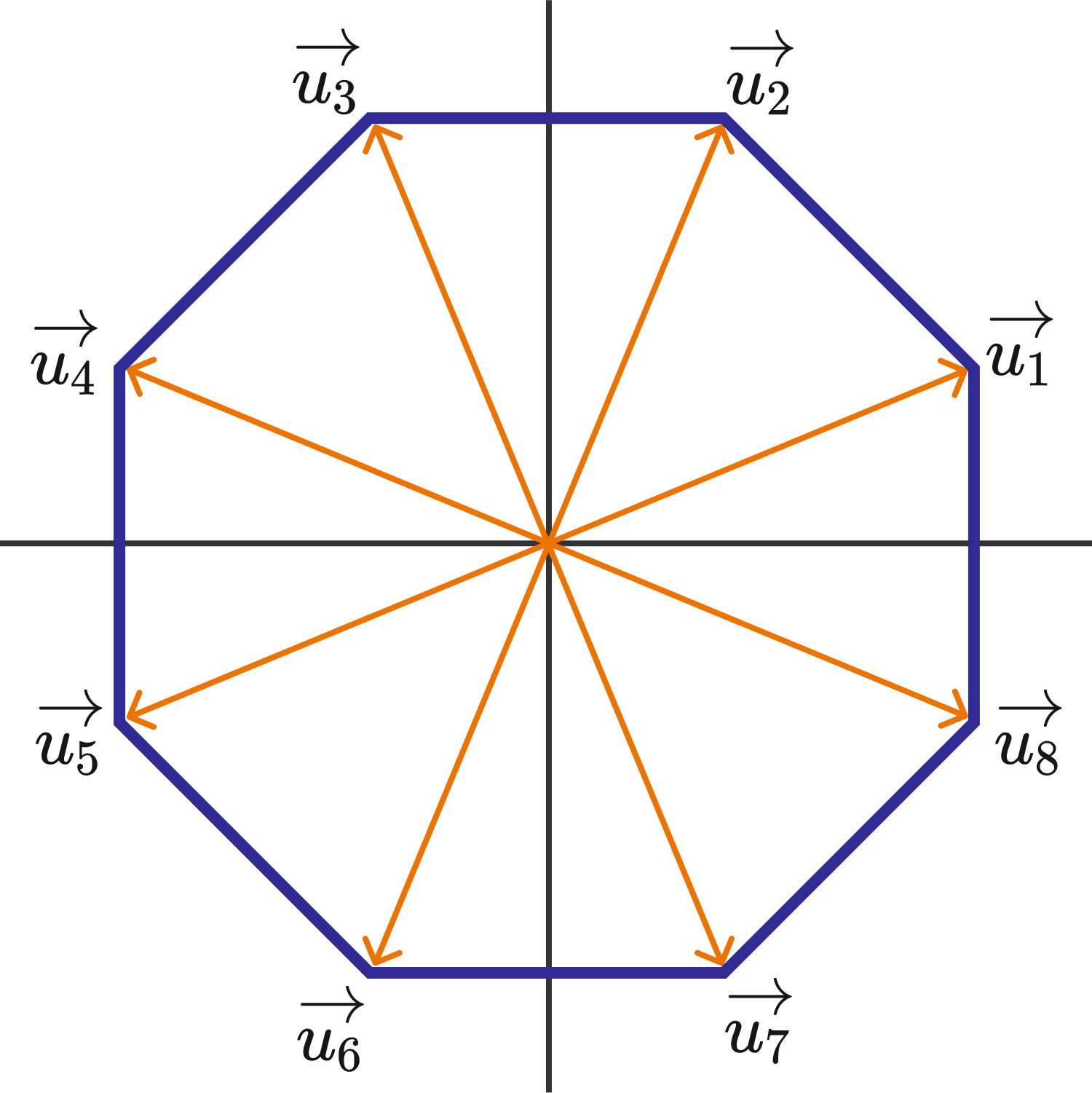# Cross Products Around the OctagonConsider the regular octagon centered at the origin as shown at right. Eight unit vectors are drawn from the center of the octagon to each of its vertices and labeled in the figure. For each pair of distinct unit vectors $\vec{u}_i, \vec{u}_j$ with $i < j$, their cross product is computed.

What is the sum of all of these cross products?

×